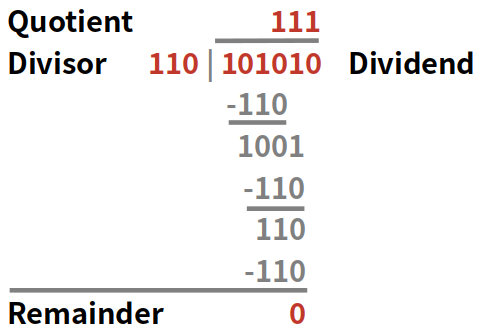# Binary Division Calculator

Created by Philip Maus
Reviewed by Steven Wooding
Last updated: Mar 23, 2022

The binary division calculator explains how to divide binary numbers. This article outlines the concepts of binary and decimal numbers and provides step-by-step instructions to carry out the division of binary numbers.

## How to divide binary numbers?

From a very early age, we are all taught to be familiar with the decimal system, numbers of the base 10. We know how to understand them and perform arithmetic operations with them. But all communication in our digital world is based on binary numbers, whose digits can only have two values: 0 and 1. These binary numbers can be converted to the decimal system, both when integer and when they are binary fraction, but arithmetic operations like addition, multiplication, or subtraction can also be executed in the binary system.

Binary division strongly follows the decimal long division. The procedure consists of repeated binary subtraction steps.

## What are the rules of binary division?

Beginning with the left (most significant) bit, it is inspected if the divisor can be subtracted from the dividends' current digit. If so, a 1 is noted in that bit of the quotient; if not, a 0. The remainder of the division process is carried, and the dividend's next digit is added to it. You repeat this procedure is until the right (least significant) bit is reached.

## How to calculate the division remainder?

The division remainder is the remainder after the last division process. It is smaller than the divisor and can therefore not be divided any further.

Let's take a look at this procedure with the example below. The binary number 101010 is divided by 110 (42 and 6 in the decimal system):We will go through the division process step by step, bit by bit:

1. We take the first bit of the dividend, which is 1. This is smaller than the divisor 110, so this bit in the quotient is 0, and the remainder of 1 is kept.
2. The next digit of the dividend is added, but 10 is still smaller than the divisor 110, so the quotient bit is 0.
3. Add the next dividend bit: 101. This is still smaller than the divisor 110; therefore, the quotient bit is 0.
4. The next dividend digit is added: 1010. This is bigger than the divisor, so we subtract the 110 from 1010, which leaves a remainder of 100. The quotient bit is set to 1.
5. The dividend bit is added to the remainder, which results in 1001. This is bigger than 110; subtract the divisor from it, which leaves 110 as a remainder. The corresponding quotient bit is 1.
6. The last dividend bit is added, and we subtract 110 from 110, which leaves a remainder of 0 and sets the last quotient bit to 1.
7. The final result is the quotient 000111 and the remainder 0.

## How do I do binary division for negative values?

Negative binary values can be managed with the two's complement signed representation. After this binary number transformation, the division of binary numbers can be executed in the same way as for positive values.

The binary division calculator makes use of the two's complement representation. This means that, for example, for an 8-bit representation, the first bit of your input numbers will be the signed bit. Consider this in your calculations, and if needed, please increase the bit representation.

Besides those basic arithmetic operations, binary numbers also allow bitwise operations, which cannot be performed with the decimal system. Those include bit shifts as well as AND, OR, and XOR operations.

## How to use the binary division calculator?

You learned about the difference between the binary and decimal system and how to divide binary numbers. Now let's understand how this binary division calculator works. For this purpose, let us see if we did everything right in the step-by-step procedure and use the same values: 101010 divided by 110.

1. Choose your binary representation. This setting defines the number of bits your input dividend, divisor, and quotient can have.
• Our dividend has 6 significant bits, so we are alright with the 8-bit representation.
1. Input your numbers:
• Dividend: 101010
• Divisor: 110
1. The binary division calculator presents your quotient and remainder in the binary and decimal system. These are the results of your division of binary numbers.
• Quotient: binary: 111, decimal: 7
• Remainder: binary and decimal: 0

In case your binary result has a value of 1 on the most significant bit and could be understood as a positive result in unsigned notation or a negative result in signed notation, both results will be displayed.

## FAQ

### How to do binary division using shifting?

Binary division, especially with divisors which are a power of 2, can be done using bit shifting to the right. A division by 2 is a shift by one bit, 4 equals 2 bits, 8 is a 3-bit shift, etc. Due to its mathematical efficiency, this method is commonly used in digital applications.

Philip Maus
Binary representation
8-bit
You can write binary numbers with no more than 8 digits. You don't have to input leading zeros.
Dividend
Divisor
People also viewed…

### Alien civilization

The alien civilization calculator explores the existence of extraterrestrial civilizations by comparing two models: the Drake equation and the Astrobiological Copernican Limits👽

### Center of a circle

The center of a circle calculator finds a circle's center using the parameters of the equation describing the circle.

### Pizza size

This calculator will help you make the most delicious choice when ordering pizza.

### Torus volume

You can determine the volume of a torus using the torus volume calculator.